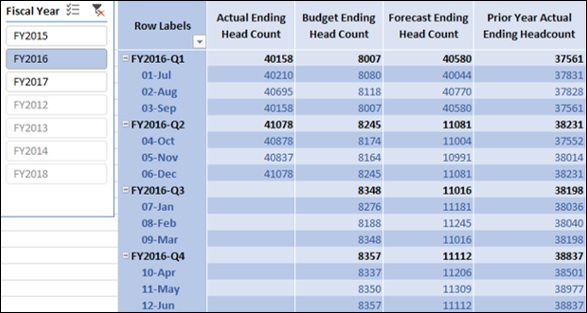You can create Ending Headcount measures for a specific period of time. The Ending Headcount is the sum of the people as on the last date in the specified period for which we have a non-blank sum of people.

The Ending Headcount is obtained as follows −

• For a Month − Sum of People at the end of the specific Month.

• For a Quarter − Sum of People at the end of the last Month of the specific Quarter.

• For a Year − Sum of People at the end of the last Month of the specific Year.

### Creating Actual Ending Headcount Measure

You can create Actual Ending Headcount measure as follows −

Actual Ending Head Count:=CALCULATE(SUM('Finance Data'[Actual People]),LASTNONBLANK('Finance Data'[Date], IF(CALCULATE(SUM('Finance Data'[Actual People]), ALL(Accounts))=0, BLANK(), CALCULATE(SUM('Finance Data'[Actual People]), ALL(Accounts)))), ALL(Accounts))

DAX LASTNONBLANK function as used above returns the last date for which you have a non-blank sum of people so that you can calculate the sum of people on that date.

### Creating Budget Ending Headcount Measure

You can create Budget Ending Headcount measure as follows −

Budget Ending Head Count: = CALCULATE(SUM('Finance Data'[Budget People]),LASTNONBLANK('Finance Data'[Date], IF(CALCULATE(SUM('Finance Data'[Budget People]), ALL(Accounts))=0, BLANK(), CALCULATE(SUM('Finance Data'[Budget People]), ALL(Accounts)))), ALL(Accounts))

### Creating Forecast Ending Headcount Measure

You can create Forecast Ending Headcount measure as follows −

Forecast Ending Head Count:= CALCULATE(SUM('Finance Data'[Forecast People]), LASTNONBLANK('Finance Data'[Date], IF(CALCULATE(SUM('Finance Data'[Forecast People]), ALL(Accounts))=0, BLANK(),CALCULATE(SUM('Finance Data'[Forecast People]), ALL(Accounts)))), ALL(Accounts))

### Creating Prior Year Actual Ending Headcount Measuree

You can create Prior Year Actual Ending Headcount measure as follows −

## Analyzing Data with Ending Headcount Measures

Create a Power PivotTable as follows −

• Add the fields Fiscal Year and Month from the Date table to Rows.